Courses

# Theory - To Show that the Area of a Triangle is half the product of the Base and the Height, Math Class 9 Notes | EduRev

## Mathematics (Maths) Class 9

Created by: Indu Gupta

## Class 9 : Theory - To Show that the Area of a Triangle is half the product of the Base and the Height, Math Class 9 Notes | EduRev

The document Theory - To Show that the Area of a Triangle is half the product of the Base and the Height, Math Class 9 Notes | EduRev is a part of the Class 9 Course Mathematics (Maths) Class 9.
All you need of Class 9 at this link: Class 9

Objective:

To show that the area of a triangle is half the product of the base and the height.

Theory:

Triangle: A triangle is one of the basic shapes in geometry: a polygon with three corners or vertices and three sides or edges which are line segments.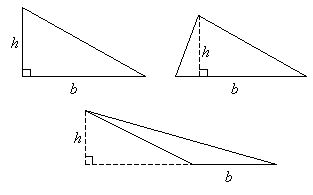Types of triangles: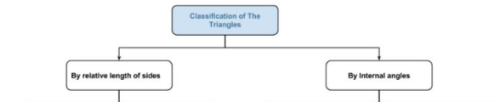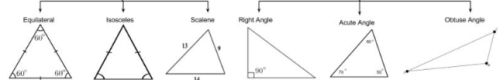Right angled triangle: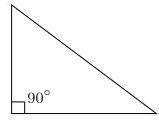A right angled triangle has one of its interior angles measuring 90°. The side opposite to the right angle is the hypotenuse; it is the longest side of the triangle. For  a right angled triangle with sides a, b, c where c is the hypotenuse, a2 + b2 = c2.

Acute angled triangle: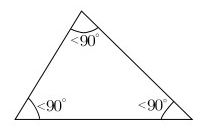A triangle that has all interior angles measuring less than 90° is an acute triangle or acute-angled triangle. For  an acute-angled triangle with sides a, b, c where  c is the greatest side,   a2 + b2 > c2.

Obtuse angled triangle: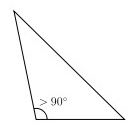A triangle that has one interior angle that measures more than 90° is an obtuse triangle or obtuse-angled triangle. For  an obtuse-angled triangle with sides a, b, c where  c is the greatest side, a2 + b2 < c2.

## Mathematics (Maths) Class 9

190 videos|232 docs|82 tests

,

,

,

,

,

,

,

,

,

,

,

,

,

,

,

,

,

,

,

,

,

,

,

,

;